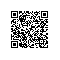# ES6：变量的解构赋值

1、数组的解构赋值

ES6允许按照一定模式，从数组和对象中提取值，对变量进行赋值，这被称为解构（Destructuring）。

 1 2 3 var a = 1; var b = 2; var c = 3;

ES6允许这样写：

 1 2 3 4 var [a,b,c] = [1,2,3]; console.log(a); //1 console.log(b); //2 console.log(c); //3

 1 2 3 4 5 6 7 8 9 10 11 12 13 14 15 16 17 18 19 20 let[foo, [[bar],[baz]]] = [1, [,]]; console.log(foo); //1 console.log(bar); //2 console.log(baz); //3   let[,two,] = [,'second',]; console.log(two); //second   let[x,y] = [4,5,6]; console.log(x); //4 console.log(y); //5   let[head, ...foot] = [1,2,3,4] console.log(head); //1 console.log(foot); //[2,3,4]   let[i1,i2,...ix] =; console.log(i1); //6 console.log(i2); //undefined console.log(ix); //[]

 1 2 3 4 5 6 var [foo2]=[]; console.log(foo2); //undefined   var [bar3,foo3]=; console.log(bar3); //1 console.log(foo3); //undefined

 1 2 3 4 5 6 7 8 let[x,y]=[1,2,3]; console.log(x); //1 console.log(y); //2   let[a,[b],c] = [1,[2,3,4],5]; console.log(a); //1 console.log(b); //2 console.log(c); //5

 1 2 3 4 5 6 7 let[a]=1; let[b]=false; let[c]=NaN; let[d]=undefined; let[e]=null; let[f]={}; console.log(a+b+c+d+e+f); //Uncaught TypeError: undefined is not a function

 1 2 3 var [v1, v2, ..., vN ] = array; let [v1, v2, ..., vN ] = array; const [v1, v2, ..., vN ] = array;

 1 2 3 4 let[x,y,z]=new Set(['a','b','c']); console.log(x); //a console.log(y); //b console.log(z); //c

 1 2 3 4 5 6 7 8 9 10 11 function* fibs(){     var a=0;     var b=1;     while(true){         yield a;         [a,b]=[b,a+b];     } } var[first,second,third,fourth,fifth,sixth]=fibs(); console.log(fifth); //3 console.log(sixth); //5

 1 2 3 4 5 6 7 8 9 10 var [foo=false]=[]; console.log(foo); //false   var [x,y='b']=['a']; console.log(x); //a console.log(y); //b   var [j,k='b']=['a',undefined]; console.log(j); //a console.log(k); //b

 1 2 3 4 5 var [x=1] = [undefined]; console.log(x); //1   var [y=1] = [null]; console.log(y); //null

 1 2 3 4 5 6 function f(){     console.log('aaa'); } let[x=f()] = ;   console.log(x); //1
 1 2 3 4 5 6 7 function f(){     console.log('aaa'); } let[x=f()] = ;   f(); //aaa console.log(x); //1

 1 2 3 4 5 6 let x; if ( === undefined) {     x = f(); } else {     x = ; }

 1 2 3 4 5 6 7 8 9 10 11 12 13 let[a=1, b=a] =[]; console.log(a); //1 console.log(b); //1   let[a=1, b=a] =; console.log(a); //2 console.log(b); //2   let[a=1, b=a] =[2,3]; console.log(a); //2 console.log(b); //3   let[a=b, b=1] =[];  //Uncaught ReferenceError: b is not defined

2、对象的解构赋值

 1 2 3 var {a,b} = {a:'apple', b:'banana'}; console.log(a); //apple console.log(b); //banana

 1 2 3 4 5 6 var {b,a} = {a:'apple', b:'banana'}; console.log(a); //apple console.log(b); //banana   var {c} = {a:'apple', b:'banana'}; console.log(c); //undefined

 1 2 3 4 5 6 7 var {a:c} = {a:'apple', b:'banana'}; console.log(c); //apple   let obj = {first:'hello', last:'world'}; let {first:f, last:l} = obj; console.log(f); //hello console.log(l); //world

 1 var { foo: foo, bar: bar } = { foo: "aaa", bar: "bbb" };

 1 2 3 4 5 var {v:n} = {v:'vue', r:'react'}; console.log(n); //vue console.log(v); //Uncaught ReferenceError: v is not defined   //console.log(r); //Uncaught ReferenceError: r is not defined

 1 2 3 4 5 6 7 8 9 10 11 let foo; let {foo} = {foo:1}; //Uncaught SyntaxError: Identifier 'foo' has already been declared   let b; let {a:b} = {a:1}; //Uncaught SyntaxError: Identifier 'b' has already been declared     let a; let {a:b} = {a:1}; console.log(a); //undefined console.log(b); //1

 1 2 3 4 5 6 7 let foo; ({foo} = {foo:1}); console.log(foo); //1   let baz; ({bar:baz}={bar:1}); console.log(baz); //1

 1 2 3 4 5 6 7 8 var obj ={     p:[         'hello', {w:'world'}     ] }; var {p:[h,{w}]} = obj; console.log(h); //hello console.log(w); //world

 1 2 3 4 5 6 7 8 9 10 11 12 var node ={     loc:{         start:{             line:1,             column:5         }     } } var {loc:{start:{line}}} = node; console.log(line); //1 console.log(loc); //Uncaught ReferenceError: loc is not defined console.log(start); //Uncaught ReferenceError: start is not defined

 1 2 3 4 5 let obj = {}; let arr = []; ({foo:obj.prop, bar:arr} = {foo:123, bar:true}); console.log(obj); //Object {prop: 123} console.log(arr); //[true]

 1 2 3 4 5 6 7 8 9 10 11 12 13 14 15 16 17 var {x=3} = {x:undefined}; console.log(x); //3   var {x, y=5} = {x:1}; console.log(x); //1 console.log(y); //5   var {x:y=5} = {}; console.log(y); //5 console.log(x); //Uncaught ReferenceError: x is not defined   var {x:y=5} = {x:10}; console.log(y); //10 console.log(x); //Uncaught ReferenceError: x is not defined   var {message: msg='Hello World!'} = {}; console.log(msg); //Hello World!

 1 2 3 4 5 var {x=3} = {x:undefined}; console.log(x); //3   var {x=3} = {x:null}; console.log(x); //null

 1 2 var {foo} = {bar: 'baz'}; console.log(foo); //undefined

 1 2 var {foo: {bar}} = {baz:'baz'}; console.log(bar); //Uncaught TypeError: Cannot match against 'undefined' or 'null'.

 1 2 var _tmp = {bar:'baz'}; console.log(_tmp.foo.bar); //Uncaught TypeError: Cannot read property 'bar' of undefined

 1 2 3 // 错误的写法 var x; {x} = {x: 1};// SyntaxError: syntax error

 1 2 // 正确的写法 ({x} = {x: 1});

 1 2 3 ({} = [true, false]); ({} = 'abc'); ({} = []);

 1 let { log, sin, cos } = Math;

 1 2 3 4 var arr = [1,2,3]; var {0:first, [arr.length-1]:last} = arr; console.log(first); //1 console.log(last);  //3

3、字符串的解构赋值

 1 2 const [a,b,c,d,e] = 'hello'; console.log(a+b+c+d+e); //hello

 1 2 let{length:len} = 'hello'; console.log(len); //5

4、数值和布尔值的解构赋值

 1 2 3 4 5 let {toString: s} = 123; console.log(s === Number.prototype.toString)// true   let {toString: s} = true; console.log(s === Boolean.prototype.toString); // true

 1 2 let { prop: x } = undefined; // TypeError let { prop: y } = null; // TypeError

5、函数参数的解构赋值

 1 2 3 4 function add([x,y]){     console.log(x+y); } add([1,2]); //3

 1 [[1, 2], [3, 4]].map(([a, b]) => a + b);  // [ 3, 7 ]

 1 2 3 4 5 6 7 function move({x=0, y=0}={}){     console.log([x,y]); } move({x: 3, y: 8}); //[3, 8] move({x: 3});  //[3, 0] move({});  //[0, 0] move();  //[0, 0]

注意，下面的写法会得到不一样的结果。

 1 2 3 4 5 6 7 function move({x, y}={x:0, y:0}){     console.log([x,y]); } move({x: 3, y: 8}); //[3, 8] move({x: 3});  //[3, undefined] move({});  //[undefined, undefined] move();  //[0, 0]

undefined会触发函数参数的默认值。

 1 [1, undefined, 3].map((x = 'yes') => x);  // [ 1, 'yes', 3 ]

6、圆括号问题

（1）变量声明语句中，不能带有圆括号。

 1 2 3 4 5 6 7 8 9 // 全部报错 var [(a)] = ;   var {x: (c)} = {}; var ({x: c}) = {}; var {(x: c)} = {}; var {(x): c} = {};   var { o: ({ p: p }) } = { o: { p: 2 } };

（2）函数参数中，模式不能带有圆括号。

 1 2 // 报错 function f([(z)]) { return z; }

（3）赋值语句中，不能将整个模式，或嵌套模式中的一层，放在圆括号之中。

 1 2 3 // 全部报错 ({ p: a }) = { p: 42 }; ([a]) = ;

 1 2 // 报错 [({ p: a }), { x: c }] = [{}, {}];

 1 2 3 [(b)] = ; // 正确 ({ p: (d) } = {}); // 正确 [(parseInt.prop)] = ; // 正确

7、用途

（1）交换变量的值

 1 [x, y] = [y, x];

（2）从函数返回多个值

 1 2 3 4 5 6 7 8 9 10 11 12 13 14 15 16 // 返回一个数组   function example() {     return [1, 2, 3]; } var [a, b, c] = example();   // 返回一个对象   function example() {     return {         foo: 1,         bar: 2     }; } var { foo, bar } = example();

（3）函数参数的定义

 1 2 3 4 5 6 7 // 参数是一组有次序的值 function f([x, y, z]) { ... } f([1, 2, 3]);   // 参数是一组无次序的值 function f({x, y, z}) { ... } f({z: 3, y: 2, x: 1});

（4）提取JSON数据

 1 2 3 4 5 6 7 8 9 10 var jsonData = {     id: 42,     status: "OK",     data: [867, 5309] };   let { id, status, data: number } = jsonData;   console.log(id, status, number); // 42, "OK", [867, 5309]

（5）函数参数的默认值

 1 2 3 4 5 6 7 8 9 10 11 jQuery.ajax = function (url, {         async = true,         beforeSend = function () {},         cache = true,         complete = function () {},         crossDomain = false,         global = true,     // ... more config         }) {     // ... do stuff };

（6）遍历Map结构

 1 2 3 4 5 6 7 8 9 var map = new Map(); map.set('first', 'hello'); map.set('second', 'world');   for (let [key, value] of map) {     console.log(key + " is " + value); } // first is hello // second is world

 1 2 3 4 5 6 7 8 9 // 获取键名 for (let [key] of map) {     // ... }   // 获取键值 for (let [,value] of map) {     // ... }

（7）输入模块的指定方法

 1 const { SourceMapConsumer, SourceNode } = require("source-map");使用钉钉扫一扫加入圈子
+ 订阅